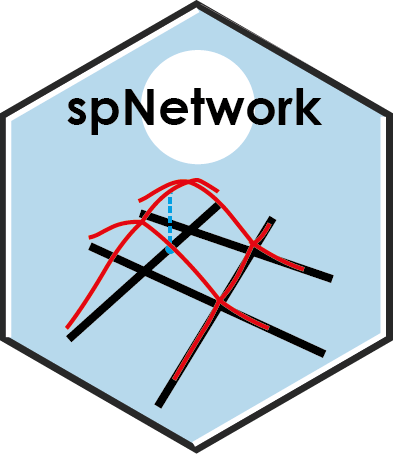# spNetworkA R package to perform spatial analysis on networks.

## Getting Started

A good start point for this package is the vignettes. They present the main features of the package

### Installing

you can install this package with the following code in R. The packages use mainly the following packages in its internal structure :

• igraph
• sp
• rgeos
• maptools
• raster
• future
• future.apply
• dplyr
• tidyR
• Rcpp
• RcppArmadillo
``devtools::install_github("JeremyGelb/spNetwork")``

### Examples

We provide here some short examples of the main features

• realizing a kernel network density estimate
``````library(spNetwork)
data(mtl_network)
data(bike_accidents)
lixels <- lixelize_lines(mtl_network,200,mindist = 50)
samples <- lines_center(lixels)
densities <- nkde(mtl_network,
events = bike_accidents,
w = rep(1,nrow(bike_accidents)),
samples = samples,
kernel_name = "quartic",
bw = 300, div= "bw",
method = "discontinuous", digits = 1, tol = 1,
grid_shape = c(1,1),
verbose=FALSE)``````
• Building a spatial matrix based on network distance and use it to calculate the Moran I with spdep.
``````library(spNetwork)
library(spdep)
data(mtl_network)

conv_function <- function(x){
if(x<=500){
return(1/x**2)
}else{
return(0)
}
}

listw <- line_ext_listw_gridded(mtl_network,maxdistance=500,
dist_func = conv_function, matrice_type='W',
grid_shape = c(5,5),
mindist = 10)

moran.test(mtl_network\$nbAccident,listw, zero.policy = T)``````

Note that you can use this in every spatial analysis you would like to perform. With the converter function of spdep (like listw2mat), you can convert the listw object into regular matrix if needed

### Work in progress

Currently, functions to calculate K-function and cross-K-function for sets of points are available but still experimental.

## Authors

• Jeremy Gelb - Creator and maintainer

## License

This project is licensed under the GPL2 License

## Acknowledgments

• Hat tip to Philippe Apparicio for its support during the development
• Hat tip to Hadley Wickham and its helpful book R packages